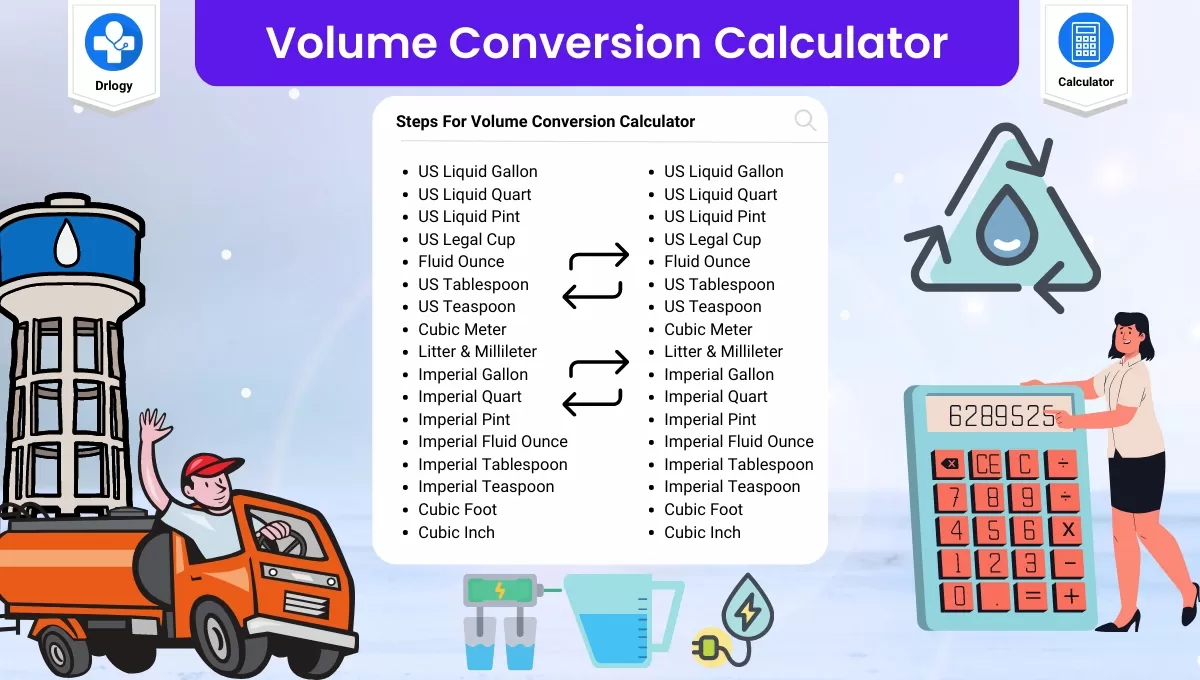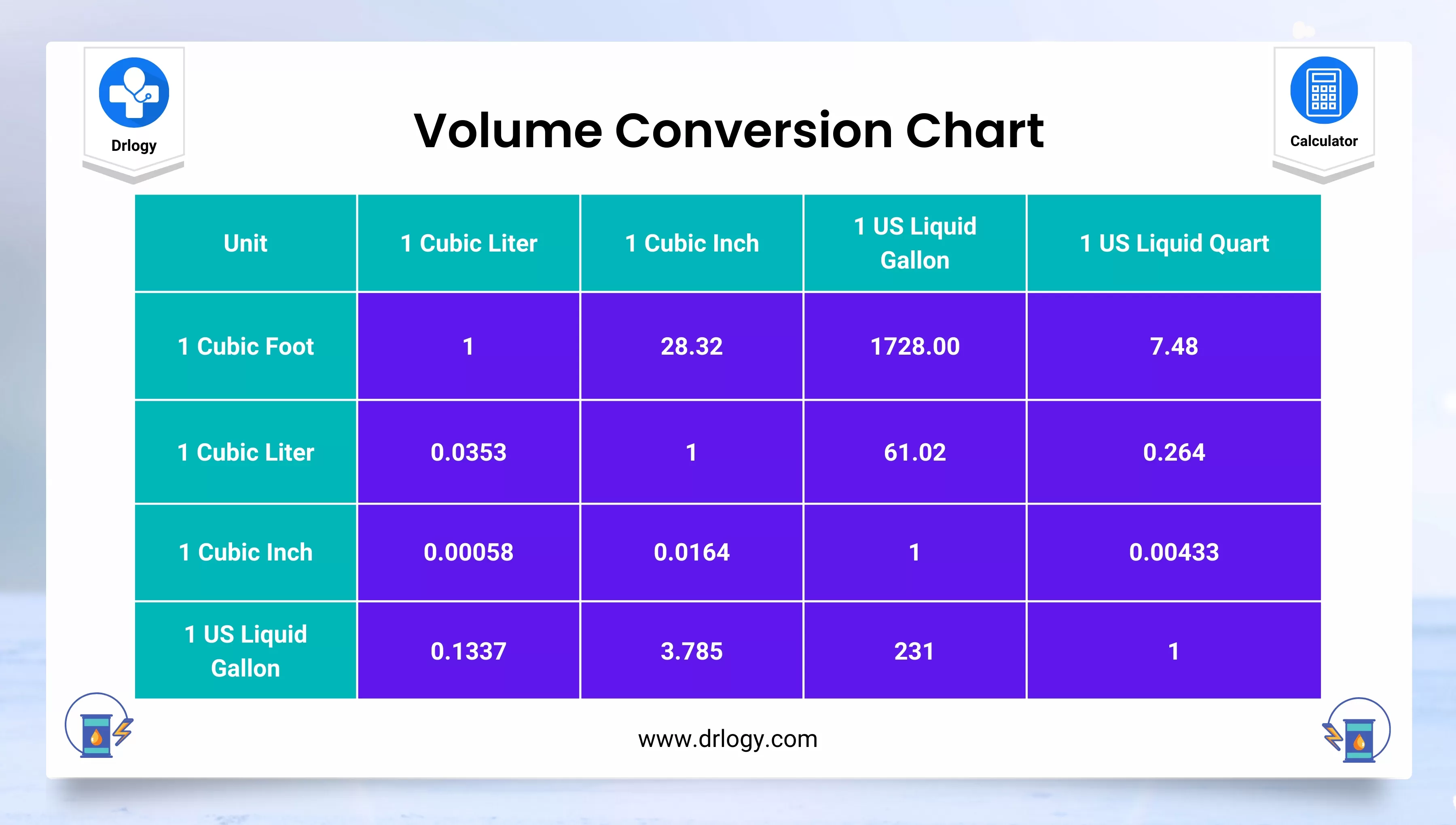• Health
• Pet
• Unit Conversion
• Medical# Volume Conversion Calculator With Formula And Chart

## Volume Conversion Calculator

Result : =
keyboard_arrow_down
=
keyboard_arrow_down

## Volume Conversion Calculator Overview

• Volume conversion calculator designed to help you easily convert between different units of volume.
• Whether you need to convert liters to gallons, cubic feet to cubic meters, or any other volume conversion, this calculator is here to help.
• To use the calculator, simply enter the value you want to convert, and select the units you are converting from. The calculator will instantly show you the converted value.
• With this easy-to-use volume conversion calculator, you'll be able to quickly and accurately convert any volume measurement you need.

## Steps For Volume Conversion Calculator

Here are the steps to use the Volume Conversion Calculator:

1. Enter the value you want to convert into the "Input" field.
2. Select the unit of measurement that the value represents from the "From" drop-down menu.
3. Select the unit of measurement that you want to convert the value to from the "To" drop-down menu.
4. The converted value will be displayed in the "Result" field.

## Volume Conversion Units

Here is a list of commonly used volume units with a brief description:

1. Milliliters (ml): A metric unit of volume equal to one-thousandth of a liter.
2. Liters (L): A metric unit of volume equal to 1000 milliliters or 1 cubic decimeter.
3. Cubic centimeters (cm³): A metric unit of volume that is equal to the volume of a cube with sides of 1 centimeter.
4. Cubic meters (m³): A metric unit of volume that is equal to the volume of a cube with sides of 1 meter.
5. Cubic inches (in³): An imperial and US customary unit of volume that is equal to the volume of a cube with sides of 1 inch.
6. Cubic feet (ft³): An imperial and US customary unit of volume that is equal to the volume of a cube with sides of 1 foot.
7. US fluid ounces (fl oz): A US customary unit of volume that is equal to 1/128th of a US gallon or 1.8047 cubic inches.
8. US cups (cup): A US customary unit of volume that is equal to 8 US fluid ounces.
9. US pints (pt): A US customary unit of volume that is equal to 2 US cups or 16 US fluid ounces.
10. US quarts (qt): A US customary unit of volume that is equal to 2 US pints or 32 US fluid ounces.
11. US gallons (gal): A US customary unit of volume that is equal to 4 US quarts or 128 US fluid ounces.

## Volume Conversion Chart

Here's a table with the most common volume units and their SI symbols:

Unit SI Symbol
Cubic Meter
Cubic Centimeter cm³
Liter L
Milliliter mL
Microliter µL
US Gallon gal
US Fluid Ounce fl oz
US Pint pt
US Quart qt

Note that the US volume units are not part of the International System of Units (SI), but they are commonly used in the United States and other countries for measuring volume in various contexts.## Volume Conversion Formula

Here are some common volume conversion formulas:

### Milliliters to Liters

• Milliliters to Liters: divide by 1000
• liters = milliliters / 1000

### Liters to Milliliters

• Liters to Milliliters: multiply by 1000
• milliliters = liters * 1000

### Cubic Centimeters to Liters

• Cubic Centimeters to Liters: divide by 1000
• liters = cubic centimeters / 1000

### Liters to Cubic Centimeters

• Liters to Cubic Centimeters: multiply by 1000
• cubic centimeters = liters * 1000

### Cubic Meters to Liters

• Cubic Meters to Liters: multiply by 1000
• liters = cubic meters * 1000

### Liters to Cubic Meters

• Liters to Cubic Meters: divide by 1000
• cubic meters = liters / 1000

### Cubic Feet to Cubic Meters

• Cubic Feet to Cubic Meters: multiply by 0.0283
• cubic meters = cubic feet * 0.0283

### Cubic Meters to Cubic Feet

• Cubic Meters to Cubic Feet: divide by 0.0283
• cubic feet = cubic meters / 0.0283

### Gallons to Liters

• Gallons to Liters: multiply by 3.7854
• liters = gallons * 3.7854

### Liters to Gallons

• Liters to Gallons: divide by 3.7854
• gallons = liters / 3.7854

These are just a few examples of conversion formulas. Depending on the units you are working with, you may need to use different formulas to convert between them.

## Volume Conversion Calculator Table

From/To Milliliters (ml) Liters (L) Cubic Centimeters (cm³) Cubic Meters (m³) Cubic Inches (in³) Cubic Feet (ft³) US Fluid Ounces (fl oz) US Cups (cup) US Pints (pt) US Quarts (qt) US Gallons (gal)
Milliliters (ml) 1 0.001 1 0.000001 0.061 0.000035 0.034 0.004 0.002 0.001 0.000264
Liters (L) 1000 1 1000 0.001 61.023 0.035 33.814 4.227 2.113 1.057 0.264
Cubic Centimeters (cm³) 1 0.001 1 0.000001 0.061 0.000035 0.034 0.004 0.002 0.001 0.000264
Cubic Meters (m³) 1,000,000 1000 1,000,000 1 61,023.7 35.3147 33,814.0 4,226.75 2,113.38 1,056.69 264.172
Cubic Inches (in³) 16.387 0.016387 16.387 0.000016387 1 0.000578704 0.554113 0.0692641 0.034632 0.017316 0.004329
Cubic Feet (ft³) 28,316.8 28.317 28,316.8 0.0283168 1728 1 957.506 119.688 59.8442 29.9221 7.48052
US Fluid Ounces (fl oz) 29.5735 0.0295735 29.5735 0.0000295735 1.805 0.00104 1 0.125 0.0625 0.03125 0.0078125
US Cups (cup) 236.588 0.236588 236.588 0.000236588 14.4375 0.00835503 8 1 0.5 0.25 0.0625
US Pints (pt) 473.176 0.473176 473.176 0.000473176 28 0.0167101 16 2 1 0.5 0.125
US Quarts (qt) 946.353 0.946353 946.353

## Volume Conversion Calculator Benefits

The benefits of using a volume conversion calculator include:

1. Accuracy: A volume conversion calculator ensures accurate conversions between different units of measurement. It eliminates the chances of human errors while converting units.
2. Time-saving: A volume conversion calculator can perform calculations quickly and saves time compared to manual calculations.
3. Convenience: The calculator is easy to use and can be accessed online or through a mobile app, making it convenient for use anywhere, anytime.
4. Multiple units: The volume conversion calculator supports multiple units of measurement, making it possible to convert between different units of volume.
5. Education: The calculator can be an educational tool for learning about different units of measurement and their conversions.
6. Professional use: The calculator is useful for professionals working in fields such as engineering, chemistry, and manufacturing, where accurate volume measurements are necessary.

Summary

Overall Volume Conversion Calculator can be useful for a wide range of audiences, including students, scientists, engineers, and anyone who works with volumes and needs to convert between different units. Check more unit conversion calculators like this to solve your daily problems on Drlogy Calculator to get exact solution.

Reference

• List of conversion factors by Wikipedia .
• Volume Conversion - Online Conversion .

favorite_border 2570 Likes

## Volume Conversion Calculator FAQ

### What is the conversion of units volume?

The conversion of units for volume can vary depending on the units being converted. Here are some common conversion factors for volume:

1. Cubic Meters to Liters: 1 cubic meter = 1000 liters
2. Liters to Milliliters: 1 liter = 1000 milliliters
3. Cubic Centimeters to Milliliters: 1 cubic centimeter = 1 milliliter
4. US Gallons to Liters: 1 US gallon = 3.78541 liters
5. US Fluid Ounces to Milliliters: 1 US fluid ounce = 29.5735 milliliters

### How do you convert volume?

To convert a volume from one unit to another, you need to know the conversion factor between the two units. Here are the general steps to convert volume:

1. Determine the volume you want to convert and it's unit.

2. Find the conversion factor for the unit you want to convert from and the unit you want to convert to.

3. Multiply the volume you want to convert by the conversion factor to get the equivalent volume in the new unit.

For example, let's say you have a volume of 3 cubic meters (m³) and want to convert it to liters (L). The conversion factor between cubic meters and liters is 1000, so you would multiply 3 m³ by 1000 to get the equivalent volume in liters:

3 m³ x 1000 = 3000 L

So 3 cubic meters is equivalent to 3000 liters.

It's important to keep track of units during conversions and ensure your final answer is in the desired unit. Additionally, be aware that there may be rounding or precision errors when converting between units.

### How do you calculate volume units?

Calculating volume units depends on the shape of the object or container being measured. Here are some common formulas for calculating volume units for different shapes:

1. Cube or Rectangular Prism: Volume (in cubic units) = Length x Width x Height

2. Cylinder: Volume (in cubic units) = π x radius^2 x height

3. Sphere: Volume (in cubic units) = (4/3) x π x radius^3

4. Cone: Volume (in cubic units) = (1/3) x π x radius^2 x height

Once you have calculated the volume in cubic units, you can convert it to the desired volume unit (e.g. liters, milliliters) using the appropriate conversion factor. For example, to convert cubic meters to liters, you would multiply the volume in cubic meters by 1000, since 1 cubic meter is equal to 1000 liters.

It's important to keep track of units during calculations and conversions to avoid errors or confusion. Additionally, be aware that rounding or precision errors may occur during calculations.

View More Health

## Medical Calculator

View More Medical

View More Pet

## Unit Conversion Calculator

View More Unit Conversion

## Financial Calculator

View More Financial
• Home >
• Calculator >
• Volume Conversion Calculator With Formula And Chart
Drlogy®
The Power To Health